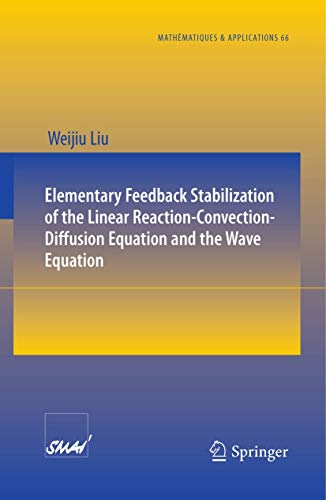# Elementary Feedback Stabilization of the Linear Reaction-Convection-Diffusion Equation and the Wave Equation: 66 (Math�matiques et Applications)

## Liu, Weijiu

0 durchschnittliche Bewertung
( 0 Bewertungen bei Goodreads )Alle Exemplare der Ausgabe mit dieser ISBN anzeigen:

Book by Liu Weijiu

Die Inhaltsangabe kann sich auf eine andere Ausgabe dieses Titels beziehen.

Cr�ticas:

From the reviews:

“This book is an introduction to the mathematical control theory of some applied partial differential equations. ... The material in this book is a nice simplification of that from the existing advanced monographs on infinite-dimensional control theory. ... This text can be used as a textbook for a one-semester graduate course on control theory for the systems governed by partial differential equations.” (Xu Zhang, Mathematical Reviews, Issue 2010 m)

“This book is an introductory text in control theory of partial differential equations (PDEs) intended for first-year graduate students in mathematics or engineering ... . book is well thought out and the topics flow together nicely. ... This textbook would be very suitable as a primary text for a course on control theory of PDEs that emphasizes application of feedback stabilization theory to concrete PDEs. ... also serve as a valuable side reference in a more general or abstract course with its many excellent examples.” (Scott W. Hansen, SIAM Review, Vol. 53 (2), 2011)

Rese�a del editor:

Unlike abstract approaches to advanced control theory, this volume presents key concepts through concrete examples. Once the basic fundamentals are established, readers can apply them to solve other control problems of partial differential equations.

��ber diesen Titel� kann sich auf eine andere Ausgabe dieses Titels beziehen.

EUR 81,31

Versand: EUR 28,99
Von Deutschland nach USA

Versandziele, Kosten & Dauer

In den Warenkorb

### Weitere beliebte Ausgaben desselben Titels

#### Vorgestellte Ausgabe

ISBN 10: 3642046258 ISBN 13: 9783642046254
Verlag: Springer, 2010
Softcover## Elementary Feedback Stabilization of the Linear Reaction-Convection-Diffusion Equation and the Wave Equation

Verlag: Springer-Verlag Gmbh Dez 2009 (2009)
ISBN 10: 3642046126 ISBN 13: 9783642046124
Neu Taschenbuch Anzahl: 1
Anbieter:
AHA-BUCH GmbH
(Einbeck, Deutschland)

Buchbeschreibung Taschenbuch. Zustand: Neu. Druck auf Anfrage Neuware -Mathematical control theory of applied partial differential equations is built on linear andnonlinearfunctionalanalysisand manyexistencetheoremsin controlt- ory result from applications of theorems in functional analysis. This makes control theoryinaccessibleto studentswhodo nothave a backgroundin functionalanalysis. Many advanced control theory books on in nite-dimensionalsystems were wr- ten, using functional analysis and semigroup theory, and control theory was p- sented in an abstract setting. This motivates me to write this text for control theory classes in the way to present control theory by concrete examples and try to m- imize the use of functional analysis. Functional analysis is not assumed and any analysis included here is elementary, using calculus such as integration by parts. The material presented in this text is just a simpli cation of the material from the existing advanced control books. Thus this text is accessible to senior undergra- ate studentsand rst-yeargraduatestudentsin appliedmathematics,who havetaken linear algebra and ordinary and partial differential equations. Elementary functional analysis is presented in Chapter 2. This material is - quired to present the control theory of partial differential equations. Since many control conceptsand theories for partial differentialequations are transplanted from nite-dimensionalcontrol systems, a brief introduction to feedback control of these systemsispresentedinChapter3.Thetopicscoveredinthischapterincludecontr- lability, observability,stabilizability, pole placement, and quadratic optimal control. 296 pp. Englisch. Artikel-Nr. 9783642046124

Neu kaufen
EUR 81,31
W�hrung umrechnen
Versand: EUR 28,99
Von Deutschland nach USA
Versandziele, Kosten & Dauer